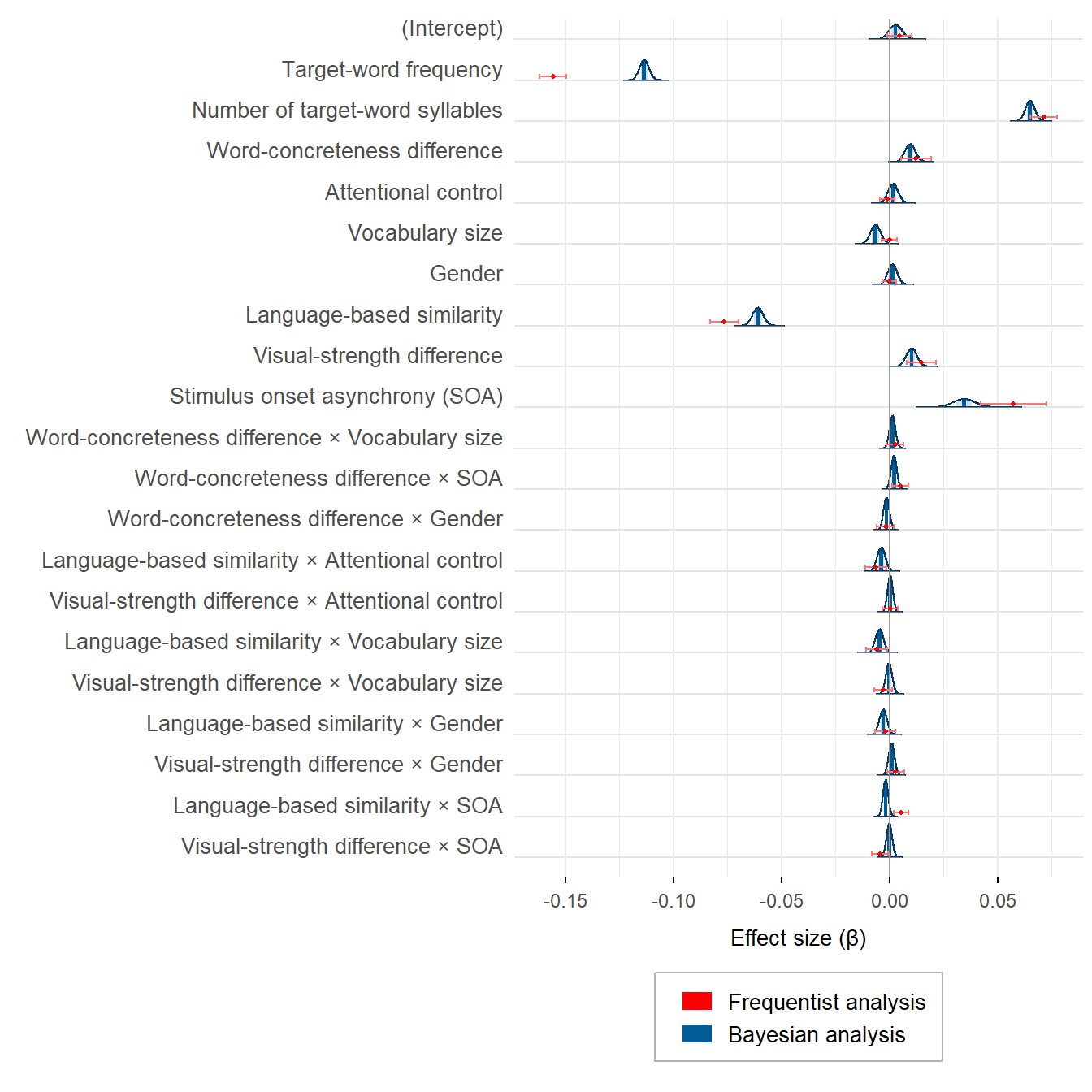# Why can't we be friends? Plotting frequentist (lmerTest) and Bayesian (brms) mixed-effects models

Frequentist and Bayesian statistics are sometimes regarded as fundamentally different philosophies. Indeed, can both methods qualify as philosophies, or is one of them just a pointless ritual? Is frequentist statistics about $$p$$ values only? Are frequentist estimates diametrically opposed to Bayesian posterior distributions? Are confidence intervals and credible intervals irreconcilable? Will R crash if lmerTest and brms are simultaneously loaded? If only we could fit frequentist and Bayesian models to the same data and plot the results together, we might get a glimpse into these puzzles.

All the analyses shown below can be reproduced using the materials at https://osf.io/gt5uf. The combination of the frequentist and the Bayesian estimates in the same plot is achieved using the following custom function from Bernabeu (2022).

### Visualising frequentist and Bayesian estimates in one plot

Both frequentist and Bayesian statistics offer the options of hypothesis testing and parameter estimation (Cumming, 2014; Kruschke & Liddell, 2018; Rouder et al., 2018; Schmalz et al., 2022; Tendeiro & Kiers, 2019, 2022; van Ravenzwaaij & Wagenmakers, 2022). In the statistical analyses conducted by Bernabeu (2022), hypothesis testing was performed within the frequentist framework, whereas parameter estimation was performed within both the frequentist and the Bayesian frameworks (for other examples of the estimation approach, see Milek et al., 2018; Pregla et al., 2021; Rodríguez-Ferreiro et al., 2020).

We’ll load the function from GitHub.

source('https://raw.githubusercontent.com/pablobernabeu/language-sensorimotor-simulation-PhD-thesis/main/R_functions/frequentist_bayesian_plot.R')
# Presenting the frequentist and the Bayesian estimates in the same plot.
# For this purpose, the frequentist results are merged into a plot from
# brms::mcmc_plot()

# install.packages('devtools')
# library(devtools)
# install_version('tidyverse', '1.3.1')  # Due to breaking changes, Version 1.3.1 is required.
# install_version('ggplot2', '5.3.5')  # Due to breaking changes, Version 5.3.5 is required.
library(tidyverse)
library(ggplot2)
library(Cairo)

# Load frequentist coefficients (estimates and confidence intervals)

KR_summary_semanticpriming_lmerTest =

confint_semanticpriming_lmerTest =

# Below are the default names of the effects
# rownames(KR_summary_semanticpriming_lmerTest$coefficients) # rownames(confint_semanticpriming_lmerTest) # Load Bayesian posterior distributions semanticpriming_posteriordistributions_weaklyinformativepriors_exgaussian = readRDS(gzcon(url('https://github.com/pablobernabeu/language-sensorimotor-simulation-PhD-thesis/blob/main/semanticpriming/bayesian_analysis/results/semanticpriming_posteriordistributions_weaklyinformativepriors_exgaussian.rds?raw=true'))) # Below are the default names of the effects # levels(semanticpriming_posteriordistributions_weaklyinformativepriors_exgaussian$data$parameter) # Reorder the components of interactions in the frequentist results to match # with the order present in the Bayesian results. rownames(KR_summary_semanticpriming_lmerTest$coefficients) =
rownames(KR_summary_semanticpriming_lmerTest$coefficients) %>% str_replace(pattern = 'z_recoded_interstimulus_interval:z_cosine_similarity', replacement = 'z_cosine_similarity:z_recoded_interstimulus_interval') %>% str_replace(pattern = 'z_recoded_interstimulus_interval:z_visual_rating_diff', replacement = 'z_visual_rating_diff:z_recoded_interstimulus_interval') rownames(confint_semanticpriming_lmerTest) = rownames(confint_semanticpriming_lmerTest) %>% str_replace(pattern = 'z_recoded_interstimulus_interval:z_cosine_similarity', replacement = 'z_cosine_similarity:z_recoded_interstimulus_interval') %>% str_replace(pattern = 'z_recoded_interstimulus_interval:z_visual_rating_diff', replacement = 'z_visual_rating_diff:z_recoded_interstimulus_interval') # Create a vector containing the names of the effects. This vector will be passed # to the plotting function. new_labels = semanticpriming_posteriordistributions_weaklyinformativepriors_exgaussian$data$parameter %>% unique %>% # Remove the default 'b_' from the beginning of each effect str_remove('^b_') %>% # Put Intercept in parentheses str_replace(pattern = 'Intercept', replacement = '(Intercept)') %>% # First, adjust names of variables (both in main effects and in interactions) str_replace(pattern = 'z_target_word_frequency', replacement = 'Target-word frequency') %>% str_replace(pattern = 'z_target_number_syllables', replacement = 'Number of target-word syllables') %>% str_replace(pattern = 'z_word_concreteness_diff', replacement = 'Word-concreteness difference') %>% str_replace(pattern = 'z_cosine_similarity', replacement = 'Language-based similarity') %>% str_replace(pattern = 'z_visual_rating_diff', replacement = 'Visual-strength difference') %>% str_replace(pattern = 'z_attentional_control', replacement = 'Attentional control') %>% str_replace(pattern = 'z_vocabulary_size', replacement = 'Vocabulary size') %>% str_replace(pattern = 'z_recoded_participant_gender', replacement = 'Gender') %>% str_replace(pattern = 'z_recoded_interstimulus_interval', replacement = 'SOA') %>% # Show acronym in main effect of SOA str_replace(pattern = '^SOA$',
replacement = 'Stimulus onset asynchrony (SOA)') %>%

# Second, adjust order of effects in interactions. In the output from the model,
# the word-level variables of interest (i.e., 'z_cosine_similarity' and
# 'z_visual_rating_diff') sometimes appeared second in their interactions. For
# better consistency, the code below moves those word-level variables (with
# their new names) to the first position in their interactions. Note that the
# order does not affect the results in any way.
sub('(\\w+.*):(Language-based similarity|Visual-strength difference)',
'\\2:\\1',
.) %>%

# Replace colons denoting interactions with times symbols
str_replace(pattern = ':', replacement = ' &times; ')

# Create plot
plot_semanticpriming_frequentist_bayesian_plot_weaklyinformativepriors_exgaussian =
frequentist_bayesian_plot(KR_summary_semanticpriming_lmerTest,
confint_semanticpriming_lmerTest,
semanticpriming_posteriordistributions_weaklyinformativepriors_exgaussian,
labels = new_labels, interaction_symbol_x = TRUE,
vertical_line_at_x = 0, x_title = 'Effect size (&beta;)',
legend_ncol = 1) +
theme(legend.position = 'bottom')

plot_semanticpriming_frequentist_bayesian_plot_weaklyinformativepriors_exgaussianFrequentist and Bayesian estimates are not so polar opposites, are they? What is more, the larger differences between some estimates are the result of the priors that were set on the corresponding effects. With uninformative priors, the frequentist and the Bayesian estimates are virtually identical.

Now it’s time to consider in earnest:

Is frequentist statistics about $$p$$ values only? Are frequentist estimates diametrically opposed to Bayesian posterior distributions? Are confidence intervals and credible intervals irreconcilable? Will R crash if lmerTest and brms are simultaneously loaded?

### Session info

If you encounter any blockers while reproducing the above analyses using the materials at https://osf.io/gt5uf, my current session info may be useful. For instance, the legend on the plot may not show if the latest versions of the ggplot2 and the tidyverse packages are used. Instead, ggplot2 3.3.5 and tidyverse 1.3.1 should be installed using install_version('tidyverse', '1.3.1') and install_version('tidyverse', '1.3.1').

sessionInfo()
## R version 4.2.2 (2022-10-31 ucrt)
## Platform: x86_64-w64-mingw32/x64 (64-bit)
## Running under: Windows 10 x64 (build 19045)
##
## Matrix products: default
##
## locale:
##  LC_COLLATE=English_United States.utf8
##  LC_CTYPE=English_United States.utf8
##  LC_MONETARY=English_United States.utf8
##  LC_NUMERIC=C
##  LC_TIME=English_United States.utf8
##
## attached base packages:
##  stats     graphics  grDevices utils     datasets  methods   base
##
## other attached packages:
##   ggtext_0.1.2        Cairo_1.6-0         forcats_0.5.2
##   stringr_1.5.0       dplyr_1.0.10        purrr_1.0.0
##  ggplot2_3.3.5       tidyverse_1.3.1     knitr_1.41
##  xaringanExtra_0.7.0
##
## loaded via a namespace (and not attached):
##   Rcpp_1.0.9       lubridate_1.9.0  assertthat_0.2.1 digest_0.6.31
##   utf8_1.2.2       plyr_1.8.8       R6_2.5.1         cellranger_1.1.0
##   ggridges_0.5.4   backports_1.4.1  reprex_2.0.2     evaluate_0.19
##  highr_0.10       httr_1.4.4       blogdown_1.16    pillar_1.8.1
##  rlang_1.0.6      uuid_1.1-0       readxl_1.4.1     rstudioapi_0.14
##  jquerylib_0.1.4  rmarkdown_2.19   labeling_0.4.2   gridtext_0.1.5
##  munsell_0.5.0    broom_1.0.2      compiler_4.2.2   modelr_0.1.10
##  xfun_0.36        pkgconfig_2.0.3  htmltools_0.5.4  tidyselect_1.2.0
##  bookdown_0.31    fansi_1.0.3      crayon_1.5.2     tzdb_0.3.0
##  dbplyr_2.2.1     withr_2.5.0      commonmark_1.8.1 grid_4.2.2
##  jsonlite_1.8.4   gtable_0.3.1     lifecycle_1.0.3  DBI_1.1.3
##  magrittr_2.0.3   scales_1.2.1     cli_3.4.1        stringi_1.7.8
##  cachem_1.0.6     farver_2.1.1     fs_1.5.2         xml2_1.3.3
##  bslib_0.4.2      ellipsis_0.3.2   generics_0.1.3   vctrs_0.5.1
##  tools_4.2.2      glue_1.6.2       markdown_1.4     hms_1.1.2
##  fastmap_1.1.0    yaml_2.3.6       timechange_0.1.1 colorspace_2.0-3
##  rvest_1.0.3      haven_2.5.1      sass_0.4.4